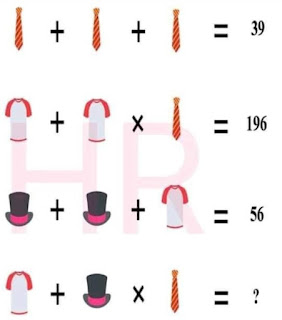Image source: Facebook

This is an interesting mathematics picture puzzle. You have 3 solved equations and from that you have to solve the fourth equation.

Find out the values of Each elements including Tie, T-shirt and Hat to solve the final equation.

Scroll down to see the correct answer below.

Explanation
Equation 1: 13 + 13 + 13 = 39  (The value of Tie = 13)
Equation 2: 14 + 14 x 13 = 196  (The value of T-shirt = 14)
Equation 3: 21 + 21 + 14 = 56  (The value of Hat = 21)

Therefore, the final equation 4 is:
=> 14 + 21 x 13

Applying BODMAS rule:
=> 14 + (21 x 13)
=> 14 + 273
=> 287